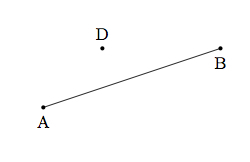# Parallelograms and Translations

Alignments to Content Standards: G-CO.C.11

Suppose $\overline{AB}$ is a line segment and $D$ is a point not on $\overline {AB}$ as pictured below:Let $C$ be the point so that $|CD| = |AB|$, $\overleftrightarrow{CD}$ is parallel to $\overleftrightarrow{AB}$, and $ABCD$ is a quadrilateral.

Draw a picture of this situation and show that $ABCD$ is a parallelogram.

## IM Commentary

The purpose of this task is to apply the definition of a parallelogram in the context of a geometric construction. The task also provides the background required to understand the mathematical definition of translation of a point by a line segment. Using the notation of this problem, if $\overline{AB}$ is a line segment then the translation of the point $D$ by segment $\overline{AB}$ can be defined in two steps:

1. If $D$ lies on $\overleftrightarrow{AB}$ then the translation of $D$ by $\overline{AB}$ is the point $C$ so that $|CD| = |AB|$ and $\overrightarrow{DC}$ intersected with $\overrightarrow{AB}$ is a ray.
2. If $D$ does not lie on $\overleftrightarrow{AB}$ then the translation of $D$ by $\overline{AB}$ is the point $C$ defined in this task. As is shown here, $C$ is the point such that quadrilateral $ABCD$ is a parallelogram.

Because the definition is so technical and the physical interpretation of translation of the plane by $\overline{AB}$ comes naturally to most students, it is not recommended to present this technical definition until students have had a lot of experience working with geometry in a formal setting: a better strategy here is to use the intuition we have about translations to build the parallelogram $ABCD$ and thereby exhibit one of the fundamental properties of translations.

The geometric construction in this task can be accomplished in several ways including

• computer technology
• patty paper
• straightedge and compass

If the latter two methods are used, then this task also illustrates G-CO.12. Patty paper has the advantage of allowing students to visually see by folding that their construction really is a parallelogram.

If the teacher wishes to give a more open ended version of this task, a different prompt would be to give the points $A, B, D$, with no restrictions if desired, and ask the students how many points $C$ there are so that $ABCD$ is a parallelogram. The work involved will be the same but the cognitive demand is higher. Even more general and challenging would be to ask how many parallelograms contain $A$, $B$, and $D$ and this has a different answer, namely 6.

Students working on this task will ''Reason Abstractly and Quantitatively'' (MP2) as they interpret the problem in terms of a picture and then use the picture to find the parallelogram in question. They will also ''Make Sense of Problems and Persevere in Solving Them'' as they put together all of the given information, and their choice of $C$, and show that the resulting shape is a parallelogram.

## Solution

Below is a picture of the given points $A, B,$ and $D$ along with the line $\ell$ parallel to $\overleftrightarrow{AB}$ through $D$:Also labeled are the points $C$ and $E$ on $\ell$ so that $\overline{CD}$, $\overline{CE}$, and $\overline{AB}$ are all congruent. Note that $ABED$ is not a quadrilateral because the diagonals $\overline{AD}$ and $\overline{BE}$ intersect so there is only one point $C$ satisfying the criteria of the problem.

We know by construction that $\overleftrightarrow{AB}$ and $\overleftrightarrow{CD}$ are parallel so to show that $ABCD$ is a parallelogram we just need to show that $\overleftrightarrow{AD}$ is parallel to $\overleftrightarrow{BC}$. Segment $\overline{AC}$ is a transverse for these lines so if $m(\angle ACB) = m(\angle CAD)$ then we can conclude that $\overleftrightarrow{AD}$ is parallel to $\overleftrightarrow{BC}$. We will show that $\triangle ACB$ is congruent to $\triangle CAD$ and this will conclude the proof that $ABCD$ is a parallelogram. We have

• $|AB| = |CD|$ by hypothesis,
• $m(\angle CAB) = m(\angle ACD)$ since these are alternate interior angles for $\overline{AC}$ which is a transverse for parallel lines $\overleftrightarrow{AB}$ and $\overleftrightarrow{CD}$,
• $\overline{AC}$ is congruent to $\overline{CA}$ since these are the same segment.
By SAS we have that $\triangle ACB$ is congruent to $\triangle CAD$ and this concludes the argument.